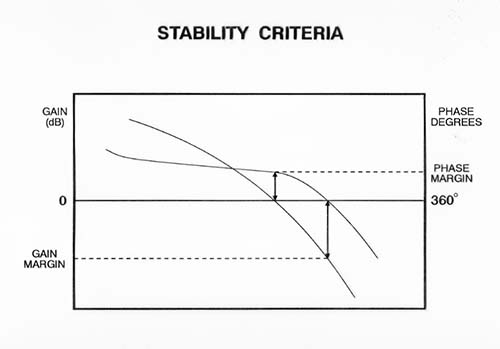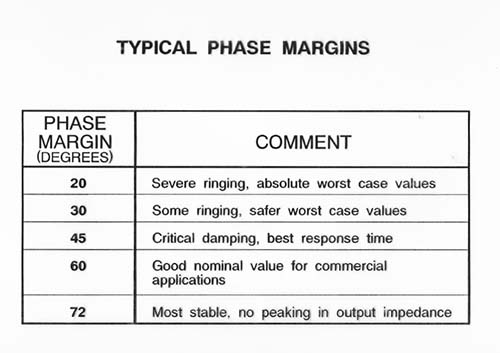# When discussing the stability of a power supply (or any system with negative feedback), we usually evaluate the phase margin as the primary indicator.  Naturally, the question arises “How much phase margin do I need?”.  The answer, as it is so often, is “It depends”.

Since the overall loop gain (closed loop performance) is expressed in terms of gain and phase, the relationship between the two is briefly reviewed.  In Figure 1 below (Bode plot), we see the gain (in dB) and the phase (in degrees) plotted over frequency.  The frequency at which the gain is 0dB (unity gain) is where we evaluate the phase margin (if the gain>0 dB or 1 when the phase reaches 360 degrees, we have positive feedback).Figure 1: Bode Plot of Gain and Phase

In the Table 1 below, one sees the effect of increasing phase margin.  At twenty degrees phase margin, the system response to a step change (in line or load) is severe overshoot/undershoot and ringing (underdamped).  As the phase margin increases, we reach a point where the system is critically damped, then overdamped.Table 1 : Phase Margin Table

So, the phase margin can be selected to meet the needs of the system.  Some power supplies need to be able to respond very quickly to step changes in load, such as the Voltage Regulator Modules for PC processors.  Other systems may be very sensitive to overshoot and ringing, so the power supply must have a phase margin that is overdamped.

Determining Gain and Phase Margins on Venable Bode Plots#### Distributed Power: What Causes These Systems to Oscillate

Distributed power systems can oscillate, especially when driving one power supply with another power supply. Download this white paper to learn what causes distributed power systems to oscillate and how you can prevent oscillation.We promise that we won't SPAM you.